# Remove First Character(s) From Left – Excel & Google Sheets

This tutorial will demonstrate how to remove the first character(s) from the left of a cell (of text) in Excel and Google Sheets.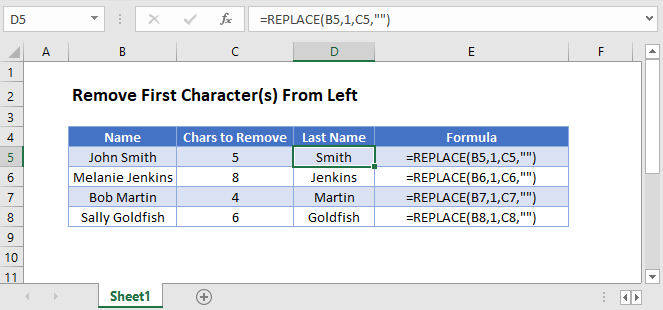## REPLACE Function

To remove characters from the left of a cell, we can use the REPLACE function to replace the first characters with an empty string (“”).

``=REPLACE(B3,1,C3,"")``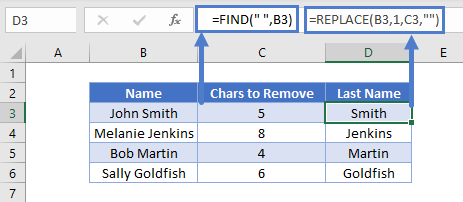The REPLACE Function allows you to define which characters to replace. In the above example, we start replacing at position 1, and the number of characters to replace is defined in column C.

## MID Function

We can also remove characters from the left of a cell by using the MID Function to return all the characters to the right of a specific position in a cell, for example, to the right of a space. We would use the SEARCH Function to find the space and then the MID Function to return the characters to the right of that space.

``=MID(B3, SEARCH(" ", B3)+1, 999)``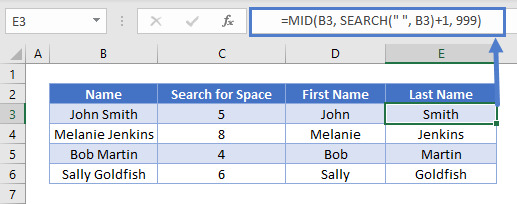We will walkthrough this below.

### SEARCH Function – Find the Space in a Cell

First, we used the SEARCH Function to find the position of the space between the first and last names.

``=SEARCH(" ", B3)``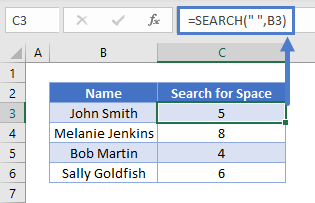We then add one onto the value returned by this formula to get the starting position of the last name.

``=SEARCH(" ",B3)+1``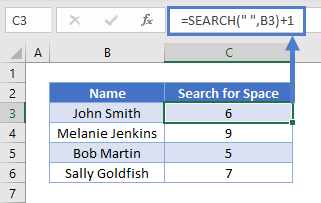### MID Function (Last Name)

Next we use the MID Function to return all the characters after the space plus 1 (the last name)

``=MID(B3, C3+1, 999)``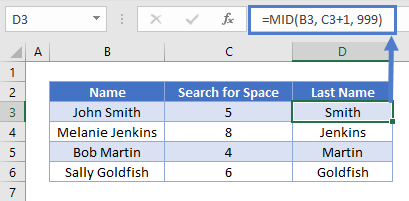Combining these 2 functions gives us the original formula for the last name.

``=MID(B3, SEARCH(B3, " ")+1, 999)``## RIGHT FUNCTION

We can also remove characters from the left of a cell by using the RIGHT Function to return a certain number of characters from the right. We use the LEN Function to count how many characters are in the cell, allowing us to remove n (ex. 1) characters from the left:

``=RIGHT(B3,LEN(B3)-C3)``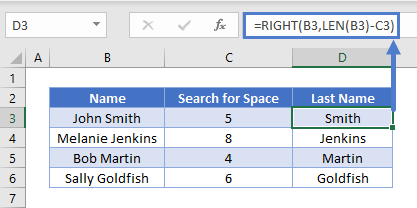We will walkthrough this below.

### LEN Function – Count Characters in a Cell

First, we will use the LEN Function to count the number of characters in the cell:

``=LEN(B3)``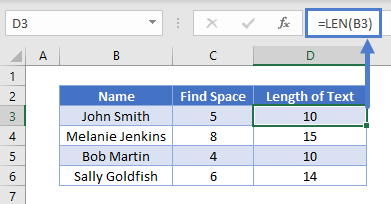In the next section, we will use the RIGHT function to trim off a certain number of characters from the left.

### Easy Excel Automation### RIGHT Function

The RIGHT Function returns a certain number of characters from the right side of a cell. For the number of characters, we will use the result of the LEN Function minus the number of characters to remove (ex. 1):

``=RIGHT(B3, D3-C3)``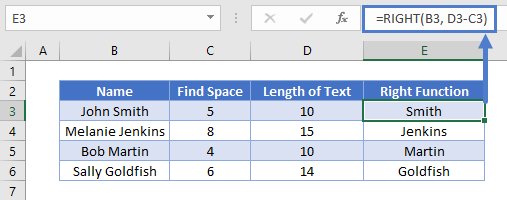Combining these functions yields the original formula.

``=RIGHT(B3,LEN(B3)-C3)``## Remove First Character(s) From Left in Google Sheets

All the examples explained above work the same in Google sheets as they do in Excel.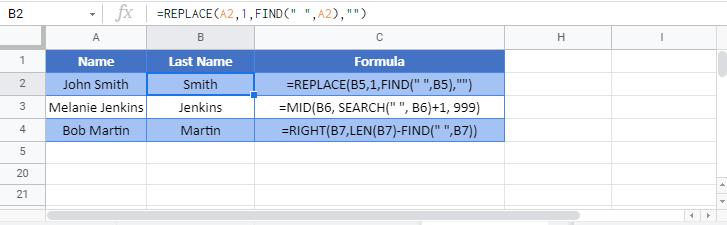### Excel Practice Worksheet

Practice Excel functions and formulas with our 100% free practice worksheets!